# 前言

DirectX11 With Windows SDK完整目录

Github项目源码

# 线程标识符与线程寻址机制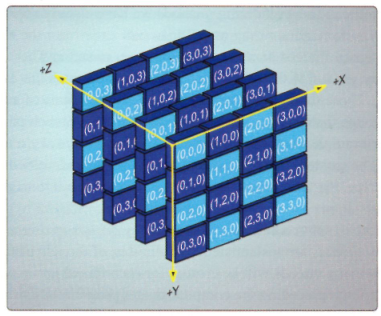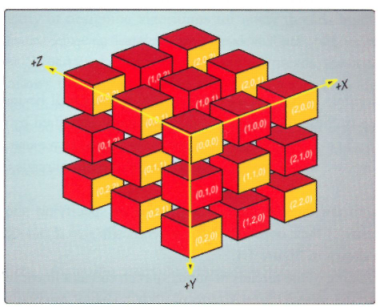SV_GroupIndex = SV_GroupThreadID.z * ThreadDimX * ThreadDimY + SV_GroupThreadID.y * ThreadDimX + SV_GroupThreadID.x;


SV_DispatchThreadID.xyz = SV_GroupID.xyz * float3(ThreadDimX, ThreadDimY, ThreadDimZ) + SV_GroupThreadID.xyz;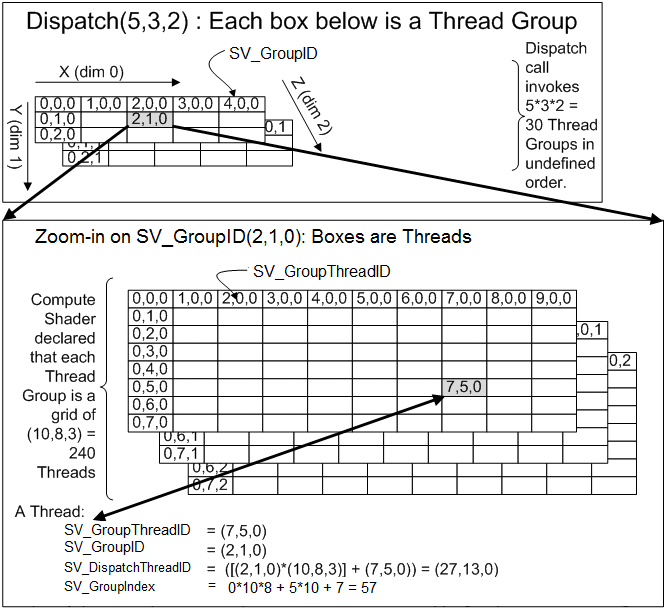# 共享内存和线程同步

groupshared float4 g_Cache;


Texture2D g_Input : register(t0);
RWTexture2D<float4> g_Output : register(u0);

groupshared float4 g_Cache;

{
// 将纹理像素值缓存到共享内存
g_Cache[GTid.x] = g_Input[DTid.xy];

// 取出共享内存的值进行计算

// 注意！！相邻的两个线程可能没有完成对纹理的采样
// 以及存储到共享内存的操作
float left = g_Cache[GTid.x - 1];
float right = g_Cache[GTid.x + 1];

// ...
}


Texture2D g_Input : register(t0);
RWTexture2D<float4> g_Output : register(u0);

groupshared float4 g_Cache;

{
// 将纹理像素值缓存到共享内存
g_Cache[GTid.x] = g_Input[DTid.xy];

// 等待所有线程完成写入
GroupMemoryBarrierWithGroupSync();

// 现在读取操作是线程安全的，可以开始进行计算
float left = g_Cache[GTid.x - 1];
float right = g_Cache[GTid.x + 1];

// ...
}


# 双调排序

## 双调序列

1. 存在一个$a_k(1 <= k <= n)$，使得$a_1 >= ... >= a_k <= ... <= a_n$成立，或者$a_1 <= ... <= a_k >= ... >= a_n$成立；
2. 序列循环移位后仍能够满足条件(1)

## Batcher归并网络

Batcher归并网络是由一系列Batcher比较器组成的，Batcher比较器是指在两个输入端给定输入值x和y，再在两个输出端输出最大值$max(x, y)$和最小值$min(x, y)$

## 双调归并网络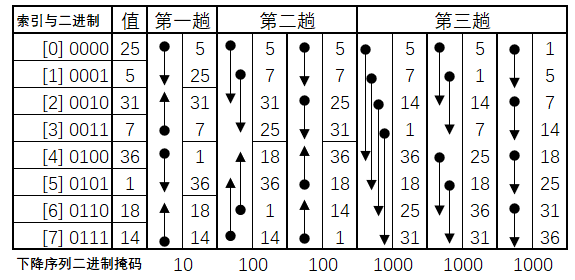1. 每一趟排序结束会产生连续的双调序列，除了最后一趟排序会产生我们所需要的单调序列
2. 对于2^k个元素的任意序列，需要进行k趟排序才能产生单调序列
3. 对于由$2^{k-1}$个元素的单调递增序列和$2^{k-1}$个元素的单调递减序列组成的双调序列，需要进行k趟交换才能产生2^k个元素的单调递增序列
4. 在第n趟排序中的第m趟交换，若两个比较数中较小的索引值为i，那么与之进行交换的数索引为$i+2^{n-m}$

## 排序核心代码实现

// BitonicSort.hlsli
Buffer<uint> g_Input : register(t0);
RWBuffer<uint> g_Data : register(u0);

cbuffer CB : register(b0)
{
uint g_Level;        // 2^需要排序趟数
uint g_MatrixWidth;  // 矩阵宽度(要求宽度>=高度且都为2的倍数)
uint g_MatrixHeight; // 矩阵高度
}



// BitonicSort_CS.hlsl
#include "BitonicSort.hlsli"

#define BITONIC_BLOCK_SIZE 512

groupshared uint shared_data[BITONIC_BLOCK_SIZE];

void CS(uint3 Gid : SV_GroupID,
uint GI : SV_GroupIndex)
{
// 写入共享数据
shared_data[GI] = g_Data[DTid.x];
GroupMemoryBarrierWithGroupSync();

// 进行排序
for (uint j = g_Level >> 1; j > 0; j >>= 1)
{
uint smallerIndex = GI & ~j;
uint largerIndex = GI | j;
bool isDescending = (bool) (g_DescendMask & DTid.x);
bool isSmallerIndex = (GI == smallerIndex);
uint result = ((shared_data[smallerIndex] <= shared_data[largerIndex]) == (isDescending == isSmallerIndex)) ?
shared_data[largerIndex] : shared_data[smallerIndex];
GroupMemoryBarrierWithGroupSync();

shared_data[GI] = result;
GroupMemoryBarrierWithGroupSync();
}

// 保存结果
g_Data[DTid.x] = shared_data[GI];
}


// 写入共享数据
shared_data[GI] = g_Data[DTid.x];
GroupMemoryBarrierWithGroupSync();


1. 找到需要与该线程对应元素进行Batcher比较的另一个元素
2. 判断当前线程对应元素与另一个待比较元素相比，是较小索引还是较大索引

uint smallerIndex = GI & ~j;
uint largerIndex = GI | j;
bool isSmallerIndex = (GI == smallerIndex);


bool isDescending = (bool) (g_DescendMask & DTid.x);


uint result = ((shared_data[smallerIndex] <= shared_data[largerIndex]) == (isDescending == isSmallerIndex)) ?
shared_data[largerIndex] : shared_data[smallerIndex];
GroupMemoryBarrierWithGroupSync();

shared_data[GI] = result;
GroupMemoryBarrierWithGroupSync();


ComPtr<ID3D11Buffer> m_pConstantBuffer;				// 常量缓冲区
ComPtr<ID3D11Buffer> m_pTypedBuffer1;				// 有类型缓冲区1
ComPtr<ID3D11Buffer> m_pTypedBuffer2;				// 有类型缓冲区2
ComPtr<ID3D11Buffer> m_pTypedBufferCopy;			// 用于拷贝的有类型缓冲区
ComPtr<ID3D11UnorderedAccessView> m_pDataUAV1;		// 有类型缓冲区1对应的无序访问视图
ComPtr<ID3D11UnorderedAccessView> m_pDataUAV2;		// 有类型缓冲区2对应的无序访问视图


void GameApp::SetConstants(UINT level, UINT descendMask, UINT matrixWidth, UINT matrixHeight);

//
// GameApp::GPUSort
//

// 按行数据进行排序，先排序level <= BLOCK_SIZE 的所有情况
for (UINT level = 2; level <= size && level <= BITONIC_BLOCK_SIZE; level *= 2)
{
SetConstants(level, level, 0, 0);
m_pd3dImmediateContext->Dispatch((size + BITONIC_BLOCK_SIZE - 1) / BITONIC_BLOCK_SIZE, 1, 1);
}


## 给更多的数据排序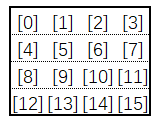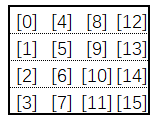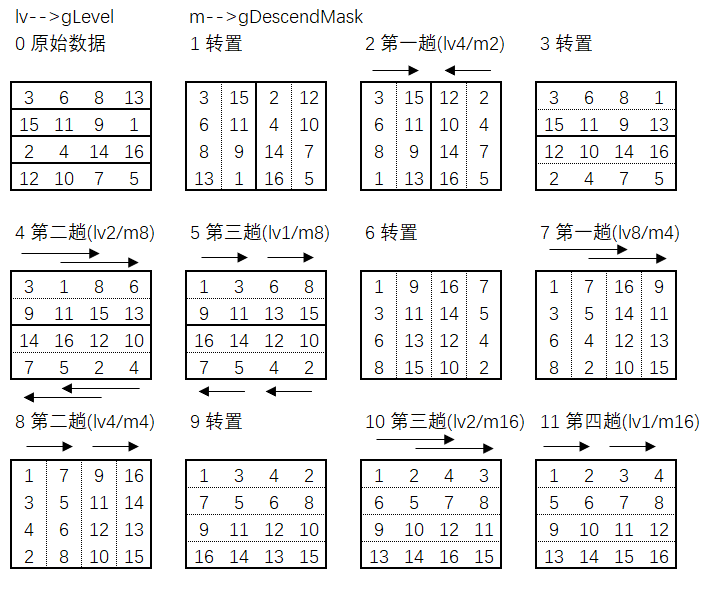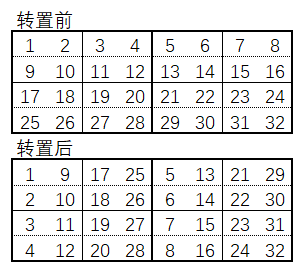// MatrixTranspose_CS.hlsl
#include "BitonicSort.hlsli"

#define TRANSPOSE_BLOCK_SIZE 16

groupshared uint shared_data[TRANSPOSE_BLOCK_SIZE * TRANSPOSE_BLOCK_SIZE];

void CS(uint3 Gid : SV_GroupID,
uint GI : SV_GroupIndex)
{
uint index = DTid.y * g_MatrixWidth + DTid.x;
shared_data[GI] = g_Input[index];
GroupMemoryBarrierWithGroupSync();

uint2 outPos = DTid.yx % g_MatrixHeight + DTid.xy / g_MatrixHeight * g_MatrixHeight;
g_Data[outPos.y * g_MatrixWidth + outPos.x] = shared_data[GI];
}


#define BITONIC_BLOCK_SIZE 512

#define TRANSPOSE_BLOCK_SIZE 16

void GameApp::GPUSort()
{
UINT size = (UINT)m_RandomNums.size();

// 按行数据进行排序，先排序level <= BLOCK_SIZE 的所有情况
for (UINT level = 2; level <= size && level <= BITONIC_BLOCK_SIZE; level *= 2)
{
SetConstants(level, level, 0, 0);
m_pd3dImmediateContext->Dispatch((size + BITONIC_BLOCK_SIZE - 1) / BITONIC_BLOCK_SIZE, 1, 1);
}

// 计算相近的矩阵宽高(宽>=高且需要都为2的次幂)
UINT matrixWidth = 2, matrixHeight = 2;
while (matrixWidth * matrixWidth < size)
{
matrixWidth *= 2;
}
matrixHeight = size / matrixWidth;

// 排序level > BLOCK_SIZE 的所有情况
for (UINT level = BITONIC_BLOCK_SIZE * 2; level <= size; level *= 2)
{
// 如果达到最高等级，则为全递增序列
if (level == size)
{
SetConstants(level / matrixWidth, level, matrixWidth, matrixHeight);
}
else
{
SetConstants(level / matrixWidth, level / matrixWidth, matrixWidth, matrixHeight);
}
// 先进行转置，并把数据输出到Buffer2
m_pd3dImmediateContext->Dispatch(matrixWidth / TRANSPOSE_BLOCK_SIZE,
matrixHeight / TRANSPOSE_BLOCK_SIZE, 1);

// 对Buffer2排序列数据
m_pd3dImmediateContext->Dispatch(size / BITONIC_BLOCK_SIZE, 1, 1);

// 接着转置回来，并把数据输出到Buffer1
SetConstants(matrixWidth, level, matrixWidth, matrixHeight);
m_pd3dImmediateContext->Dispatch(matrixWidth / TRANSPOSE_BLOCK_SIZE,
matrixHeight / TRANSPOSE_BLOCK_SIZE, 1);

// 对Buffer1排序剩余行数据
m_pd3dImmediateContext->Dispatch(size / BITONIC_BLOCK_SIZE, 1, 1);
}
}


512 0.000020 0.000003 0.000186
1024 0.000043 0.000007 0.000226
2048 0.000102 0.000009 0.000310
4096 0.000245 0.000009 0.000452
8192 0.000512 0.000010 0.000771
16384 0.001054 0.000012 0.000869
32768 0.002114 0.000012 0.001819
65536 0.004448 0.000014 0.002625
131072 0.009600 0.000015 0.005130
262144 0.021555 0.000021 0.008983
524288 0.048621 0.000030 0.015652
1048576 0.111219 0.000044 0.040927
2097152 0.257118 0.000072 0.188662
4194304 0.444995 0.000083 0.300240
8388608 0.959173 0.000124 0.465746
16777216 2.178453 0.000177 0.915898
33554432 5.869439 0.000361 2.269989

DirectX11 With Windows SDK完整目录

Github项目源码

posted @ 2019-02-19 02:37  X_Jun  阅读(1860)  评论(1编辑  收藏  举报
levels of contents